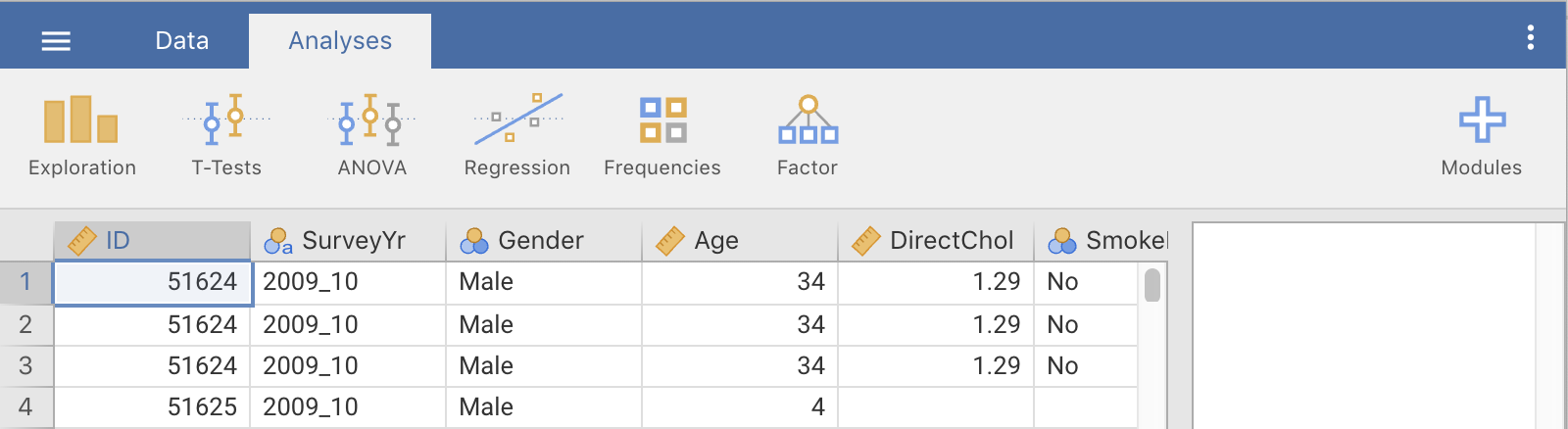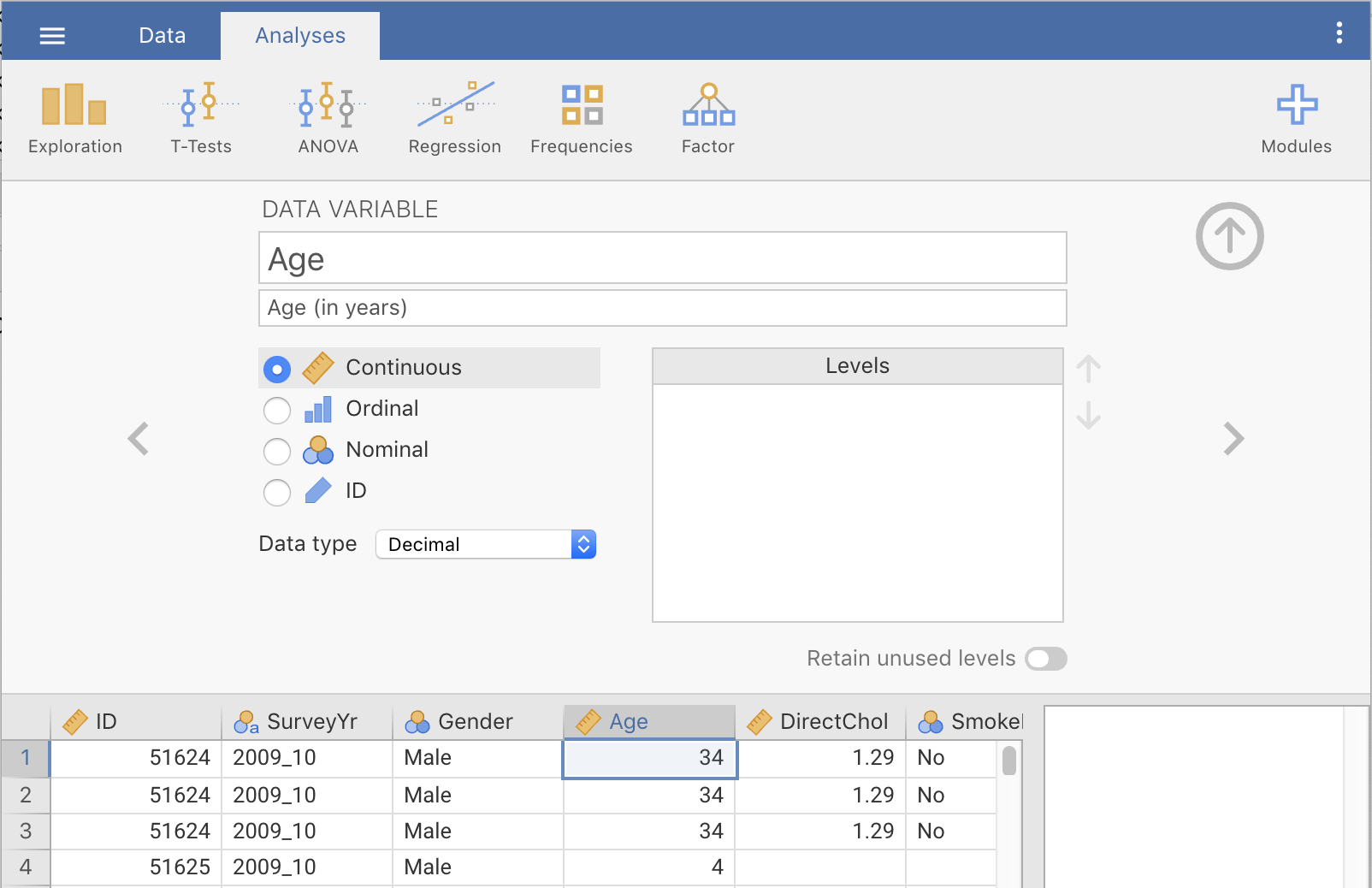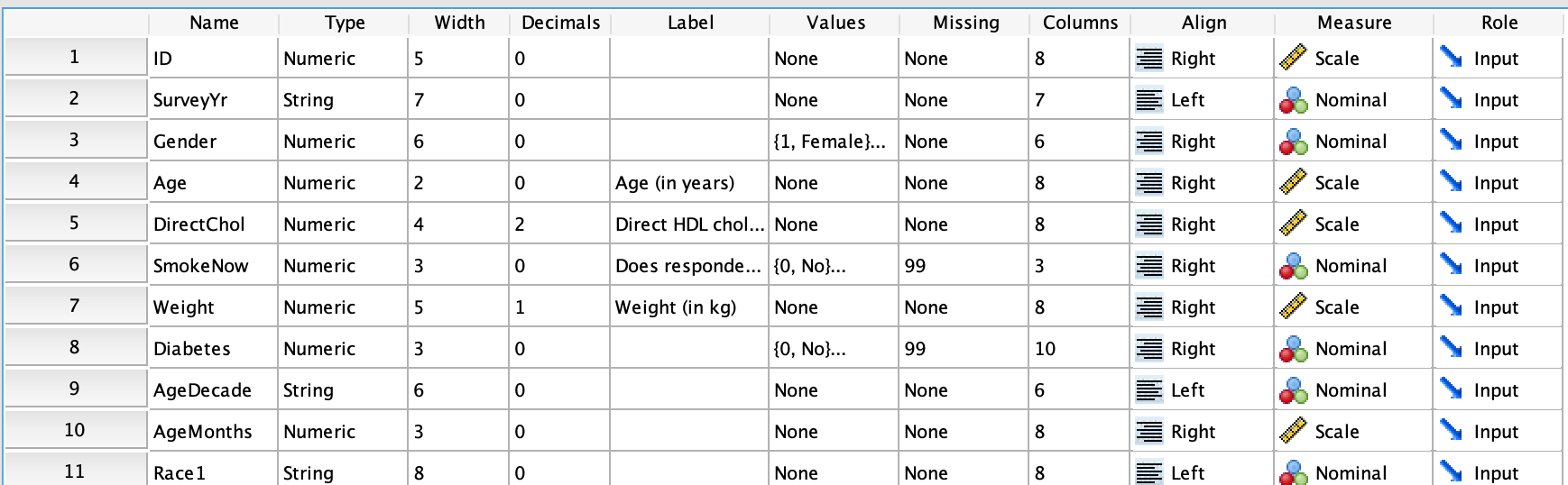## 11.2 Describing data in jamovi and SPSS

In practice, quantitative research requires the use of a computer for producing graphs and completing calculations. In this book, two statistical software packages are described for analysis of data:

(For reason to avoid Excel and other spreadsheets, read this information from earlier in this book.)

This section makes only brief notes about setting up data in these software packages; consult a comprehensive reference for more (and better) details. For both packages, however, declaring the variables correctly is very important (Table 11.1).

Practically all software, including jamovi and SPSS record data in a spreadsheet-like grid, with the variables in the columns, and the units of analysis in the rows.

TABLE 11.1: Different types of variables, and their descriptions in jamovi and SPSS
Type of variable Further classification In jamovi In SPSS
Qualitative Nominal Nominal Nominal
Ordinal Ordinal Ordinal
Quantative Discrete Continuous, Integer Scale
Continuous Continuous, Decimal Scale

### 11.2.1 Using jamovi

In jamovi, nominal variables are called Nominal, and ordinal variables are called Ordinal (Table 11.1). In jamovi, continuous quantitative variables are called continuous decimal, and discrete quantitative variables are called (confusingly) continuous integer.

To add this information to jamovi, double-click on the variable name at the top of the data worksheet (Fig. 11.1), which produces Fig. 11.2. This opens an area where the data can be described:

• Nominal qualitative variables are set as Nominal, and the levels described in the Levels area to the right
• Ordinal qualitative variables are set as Ordinal, and the levels described in the Levels area to the right.
• Quantitative continuous variables are set as Continuous with the Data type as Decimal.
• Quantitative discrete variables are set as Continuous with the Data type as Integer.

When the information has been entered, clicking the up-arrow on the top right (Fig. 11.2) closes this window.FIGURE 11.1: jamovi: The variable names at the top of the columns of dataFIGURE 11.2: jamovi: Setting the variable type

### 11.2.2 Using SPSS

In SPSS, variables are described in the Variable View window (not the Data View window). Each variable is then described in the Measure column (Fig. 11.3):

• Nominal qualitative variables are called Nominal.
• Ordinal qualitative variables are called Ordinal.
• Quantitative variables are called Scale, regardless of whether they are discrete or continuous.FIGURE 11.3: SPSS: Setting the variable type